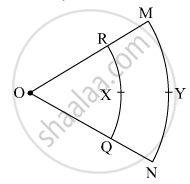# In the Given Figure, O is the Centre of the Sector. ∠ Roq = ∠ Mon = 60° . Or = 7 Cm, and Om = 21 Cm. Find the Lengths of Arc Rxq and Arc Myn. ( π = 22 7 ) - Geometry

#### Question

In the given figure, O is the centre of the sector. $\angle$ROQ =  $\angle$MON = 60° . OR = 7 cm, and   OM = 21 cm. Find the lengths of  arc RXQ and arc MYN. ($\pi = \frac{22}{7}$)#### Solution

Given: ∠ROQ = ∠MON = 60°,

radius (r) = OR = 7 cm, radius (R) = OM = 21 cm

To find: Lengths of arc RXQ and arc MYN.

i. Length of arc RXQ = θ/360 xx 2π"r"

= 60/360 × 2 × 22/7 × 7

= 1/6 × 2 × 22

= 7.33 cm

ii. Length of arc MYN = θ/360 xx 2π"R"

= 60/360 × 2 × 22/7 × 21

= 1/6 × 2 × 22 × 3

= 22 cm

∴ The lengths of arc RXQ and arc MYN are 7.33 cm and 22 cm respectively.

Is there an error in this question or solution?